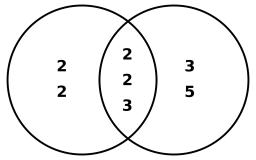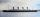# Lcm 2

Create the smallest possible number that is divisible by numbers 5,8,9,4,3

a =  360

### Step-by-step explanation:Did you find an error or inaccuracy? Feel free to write us. Thank you!Tips to related online calculators
Do you want to calculate least common multiple two or more numbers?
Do you want to perform natural numbers division - find the quotient and remainder?

## Related math problems and questions:

• Find unknown numberWhat is the number between 50 and 55 that is divisible by 2,3,6,9?
• LCM of two numberFind the smallest multiple of 63 and 147
• Counting numberWhat is the smallest counting number divisible by 2,5,7,8 and 15?
• The smallest numberWhat is the smallest number that can be divided by both 5 and 7
• LCMWhat is the least common multiple of 5, 50, 14?
• LCMCommon multiple of three numbers is 3276. One number is in this number 63 times, second 7 times, third 9 times. What are the numbers?
• PlumsIn the bowl are plums. How many would be there if we can divide it equally among 8, 10 and 11 children?
• DecomposeDecompose into primes and find the smallest common multiple n of (16,20) and the largest common divisor D of the pair of numbers (140,100)
• Divisible by nineHow many three-digit natural numbers in total are divisible without a remainder by the number 9?
• By sixFrom the digits 1,2,3,4 we create the long integer number 123412341234. .. .. , which will have 962 digits. Is this number divisible by 6?
• Reminder and quotientThere are given numbers A = 135, B = 315. Find the smallest natural number R greater than 1 so that the proportions R:A, R:B are with the remainder 1.
• A number 5A number is divisible by 24, 25, and 120 if it is increased by 20. Find the number.
• MO C–I–1 2018An unknown number is divisible by just four numbers from the set {6, 15, 20, 21, 70}. Determine which ones.
• Game roomWinner can took win in three types of jettons with value 3, 30 and 100 dollars. What is minimal value of win payable in this values of jettons?
• Lcm of three numbersWhat is the Lcm of 120 15 and 5
• Three TitanicsThree steamers sailed from the same port on the same day. The first came back on the third day, fourth 4th day and the third returned sixth day. How many days after leaving the steamers met again in the harbor?
• LCD 2The least common denominator of 2/5, 1/2, and 3/4